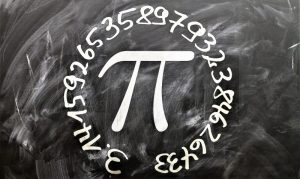Mathematics

# Irrational numbers

In the group of real numbers, there are rational and irrational numbers. These last ones cannot be expressed as a fraction and can be of two types, algebraic or transcendental. Irrational numbers are those that cannot be expressed in fractions because they contain indeterminate decimal elements and are used in complex mathematical operations such as algebraic equations and physical formulas.Related topics

## What are irrational numbers?

Irrational numbers are part of the set of real numbers that is not rational, i.e. it cannot be expressed as a fraction. This set of numbers is made up of all decimal numbers whose decimal part has infinite numbers. They are represented by the letter I or with the representation R-Q ( This is the subtraction of real numbers minus rational numbers ). They can be algebraic or transcendent.

## Which are the irrational numbers?

The set of irrational numbers is formed by algebraic numbers and transcendent numbers.

## What are they for?

They are used to perform operations in factual sciences such as physics, chemistry, mathematics among others.

## Characteristics

Of the most representative characteristics of irrational numbers we can cite the following:

• They are part of the set of real numbers.
• They can be algebraic or transcendent.
• They cannot be expressed as a fraction.
• They are represented by the letter I.
• They have infinite decimal numbers.
• It has commutative and associative properties.
• They cannot be represented as a division of two whole numbers.

## History

It is presumed that the mathematician who first identified this set of numbers was a disciple of Pythagoras called Hippasus. This personage was trying to describe the root of the number 2 in the form of a fraction and showed that there are numbers that are not rational because they cannot be expressed with fractions.

It is said that this discovery was not well accepted by Pythagoras, who stated that all numbers have perfect values. As the master Pythagoras could not deny the discovery of Hippasus, they threw Hippasus overboard of a ship and he died drowned.

There is also another version of the history of irrational numbers that comes from Ancient Greece. It states that in the practice of measuring lengths of a segment of a line that could only be fractionated, the Greeks identified numbers that could not be fractionated. This discovery is attributed to Pythagoras, in determining the existence of segments of the line that are immeasurable in relation to a segment that is taken as a unit in a measurement system.

## How are they represented?

They are represented by the capital letter I because with the lowercase i the imaginary numbers are represented. They are also usually represented in the following way R-Q (this means Real Numbers – Rational Numbers). However, it is important to mention that there are irrational numbers that have their own symbols. This is the case of the Pi number or the golden number.

## Classification of irrational numbers

Irrational numbers are classified into algebraic numbers and transcendental numbers.

Algebraic numbers are those that come from solving some algebraic equation and are finite numbers of free or nested radicals. Example: non-exact roots.

Transcendent numbers are those that come from trigonometric, logarithmic and exponential transcendent functions. These numbers are not finite numbers of free or nested radicals. Example: the number Pi =3.141592653589…; the golden number = 1,618033988749…; the number of Euler = 2,718281828459…

## Operations

The operations of addition, subtraction, multiplication and division are not well defined because when applied to irrational numbers they do not tend to result in irrational numbers. With this in mind, the following observations are important:

• If a rational number is added to an irrational number, the result will always be irrational.
• If a rational number (other than zero) is multiplied by an irrational number, the product will be irrational.
• It has commutative and associative properties.
• The multiplication is distributive in relation to the operations of addition and subtraction.
• It has its opposite or negative element that annuls it.

## Properties

Irrational numbers have the following properties:

• Switching: irrational numbers can be added or multiplied.
• Associative: they can be grouped.
• Closed: any irrational number added, subtracted, multiplied or divided will not always result in an irrational number. This is not true in the case of radication.

## Examples of irrational numbers

As an example of irrational numbers we can mention the following:

#### Examples of algebraic irrational numbers

• √7
• 0,1961325454898161376813268743781937693498749…

#### Example of transcendental irrational numbers

• Pi = 3.141592653589…
• Golden Number = 1,618033988749
• Euler number = 2,718281828459…
Written by Gabriela Briceño V.